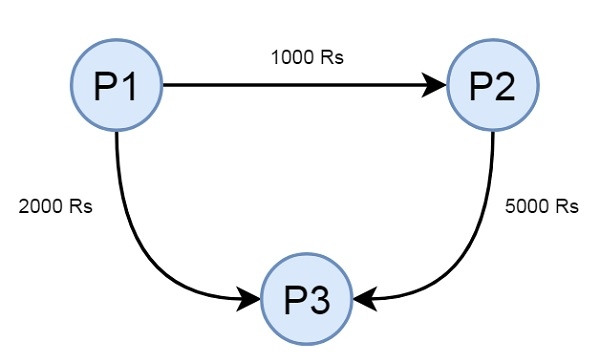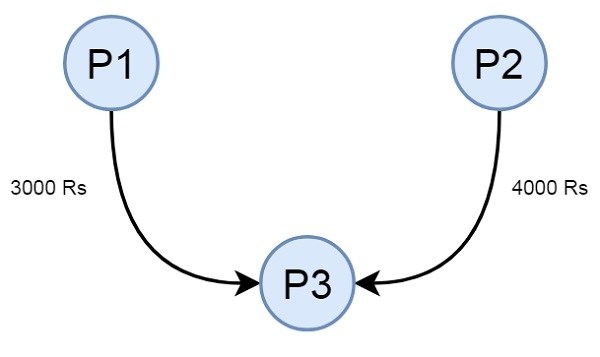# Minimize Cash Flow among a given set of friends who have borrowed money from each other in C++

Suppose there are few friends. They have borrowed money from each other. So there will be some cash flow on the network. Our task is to minimize the cash flow in the network. Suppose there are three friends P1, P2, and P3. The cash flow among them is like below −This cash flow is not minimum; we have to minimize it. Then the final diagram will be like −To solve this problem we will use the greedy approach. Here in each step settle all amounts of one person and recur for remaining n-1 persons. Now the question comes, how to choose the first person? The answer is, calculate the net amount for every person where the net amount is obtained by subtracting all debts from all credits. When the net amount is evaluated, then find two nodes which are minimum and maximum. These two persons are most creditors and debtors. The person with min value is the first person. The algorithm is like below −

• For every person Pi, from 0 to n – 1, do the following steps

• Compute the net amount for every person. Net mount for a person I can be computed by subtracting the sum of all debts from the sum of all credits.

• Find maximum creditor Pc and maximum debtor Pd. Suppose the maximum amount to be credited to a maximum creditor is max_credit, and the maximum amount debited from a maximum debtor is called max_debit.

• set x: = minimum of max_credit and max_debit. Then debit x from Pd, and credit x to Pc

• If x is the same as the max_credit, then remove Pc from the set and recur for next n-1 persons.

• if x is same as max_debit, then remove Pd from a set of persons and recur for next n-1 persons

## Example

Live Demo

#include<iostream>
#include<algorithm>
#define N 3
using namespace std;
int getMinIndex(int arr[]) {
int minInd = 0;
for (int i=1; i<N; i++)
if (arr[i] < arr[minInd])
minInd = i;
return minInd;
}
int getMaxIndex(int arr[]) {
int maxInd = 0;
for (int i=1; i<N; i++)
if (arr[i] > arr[maxInd])
maxInd = i;
return maxInd;
}
int max_credit = getMaxIndex(amount), max_debit = getMinIndex(amount);
if (amount[max_credit] == 0 && amount[max_debit] == 0)
return;
int min_val = min(-amount[max_debit], amount[max_credit]);
amount[max_credit] -= min_val;
amount[max_debit] += min_val;
cout << "P" << max_debit << " sends " << min_val << " to " << "P" << max_credit << endl;
}
void minCashFlow(int graph[][N]) {
int amount[N] = {0};
for (int p=0; p<N; p++)
for (int i=0; i<N; i++)
amount[p] += (graph[i][p] - graph[p][i]);
}
int main() {
int graph[N][N] = {
{0, 1000, 2000},
{0, 0, 5000},
{0, 0, 0},};
minCashFlow(graph);
}

## Output

P1 sends 4000 to P2
P0 sends 3000 to P2